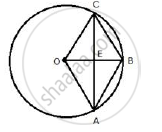Share

# Oabc is a Rhombus Whose Three Vertices A, B and C Lie on a Circle with Centre O. (Ii) If the Area of the Rhombus is 32 3 Cm2 Find the Radius of the Circle. - Mathematics

Course

#### Question

OABC is a rhombus whose three vertices A, B and C lie on a circle with centre O.

(ii) If the area of the rhombus is 32 3 cm2 find the radius of the circle.

#### SolutionArea of rhombus = 32 sqrt(3)    cm ^2

But area of rhombus OABC = 2xx  area of ΔOAB .

Area of rhombus OABC = 2xx sqrt(3)/4 r^2

Where r is the side of the equilateral triangle OAB.

2 xx sqrt(3)/4 r^2 = 32sqrt(3)

⇒  sqrt(3)/2 r^2 = 32sqrt(3)

⇒ r^2 = 64

⇒ r= 8

Therefore, radius of the circle = 8 cm

Is there an error in this question or solution?